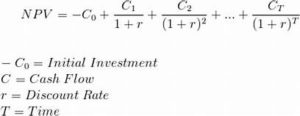# What is Net Present Value (NPV)? What are the Advantages and Disadvantages of Net Present Value?

## Introduction

Net present value method is used for evaluating the project proposals. Time value of money for cash inflows is considered. Net present value is the summation of the present value of cash inflow and the present value of cash outflow. Net present value is the difference between the total present value of future cash inflows and the total present value of future cash outflows.

## The formula of NPV Calculation• It recognizes the time value of money.
•  It considers the total benefits arising out of the proposal.
• It is the best method for the selection of mutually exclusive projects.
•  It helps to achieve the maximization of shareholders’ wealth.

• It is difficult to understand and calculate.
• It needs the discount factors for calculation of present values.
•  It is not suitable for the projects having different effective lives.

## Example of NPV Calculation

1. The initial investment for the plant is \$ 50,000. Revenue from this project is \$ 70,000 for the first two years and 50,000 for the next two years. Cost of Capital is 10%.

Requirements :

• Calculate NPV of the Project.
• Whether the project is worthwhile?

Solution :

NPV = (50,000)+70,000 x .909+70,000 x .826+50,000 x .751+50,000 x .683

= (50,000)+63,630+57,820+37,550+34,150

= 143,150

As NPV of the project is positive, the project is considered worthwhile.

* 1+r = 1/1.10 = .909

## Points to be considered

• Only Incremental cost will be considered
• Opportunity costs will be taken
• The sunk cost will be excluded
• Depreciation will not be considered
• Inflation to be incorporated when requiring
• Interest Cost will be excluded from calculating NPV as it is included in DCF factor
• If assets purchased in last day of the year, depreciation will be charged for the whole year
• If assets purchased at the beginning of the year cost will be charged on t0 and depreciation will be charged from t1
• Working capital will be incurred at the beginning of the year and realized thereafter.

## Accept/Reject criteria

If the present value of cash inflows is more than the present value of cash outflows, it would be accepted. If not, it would be rejected

## Conclusion

Net present value (NPV) is the combined present value of both the investment cash flow and the return or withdrawal cash flow. The user will use Discount Rate which is the desired rate of return of the user. This discount rate could be Real or Money i.e inflation may be included sometimes.